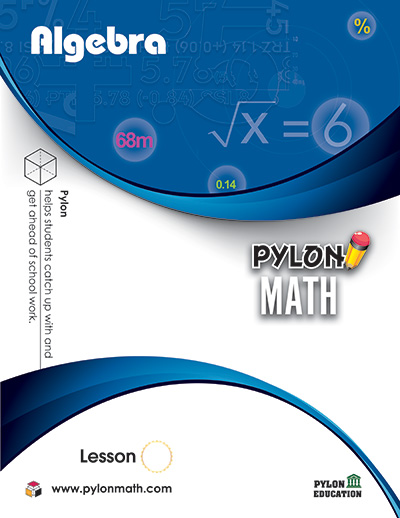# Algebra### 8th / 9th Grade

FREE TRIAL- click to view and download

1. ### Equations

• Equations, Transforming Equations
• Solving Equations for x or y
• Absolute Value Equations
2. ### Word Problems

• Writing Equations, Consecutive Integers ‘as many as’ problems
• Simple Expressions, Number Problems
• Coin Problems, Ratios
3. ### Word Problems

• Motion Problems; Same Direction
• Opposite Directions, Toward Each Other
• Work Problems; Work Rates, Joint Work
• Missing Work Rate
4. ### Percent Word Problems

• Percent of Change, Changing percents
• Finding the Original Number
• Find the New Number
• Stocks, Mixture Problems
• Dimensions
5. ### The Coordinate Planes

• Solutions of Equations, Slopes
• The Slope-Intercept Forms
• Parallel and Perpendicular Lines
• x- and y-Intercepts
6. ### Systems of Equations

• Substitution, Elimination, Combination
• Graphing Methods
• Linear Equations
• Word Problems
1. ### Inequalities

• Solving Inequalities, Compound Inequalities
• Absolute Value and Inequalities, Graphs
• Word Problems
• Systems of Linear Inequalities
2. ### Polynomials

• Adding Like Terms, Multiplying Monomials
• Powers of Monomials, Simplifying Monomials
• Simplifying Fractions, Negative Exponents
• Factoring GCF, Multiplying Polynomials
3. ### Factoring

• Factoring Trinomials
• Difference of Two Squares
• Polynomial Equations
• Factoring Polynomials
4. ### Quadratic Functions

• Functions and Relations
• Maximum or Minimum Value
• Graph, Transforming Graphs
• Vertex, Finding Zeros
• x- and y- Intercepts, x- Intercept and Vertex
• Square Roots, Inequality